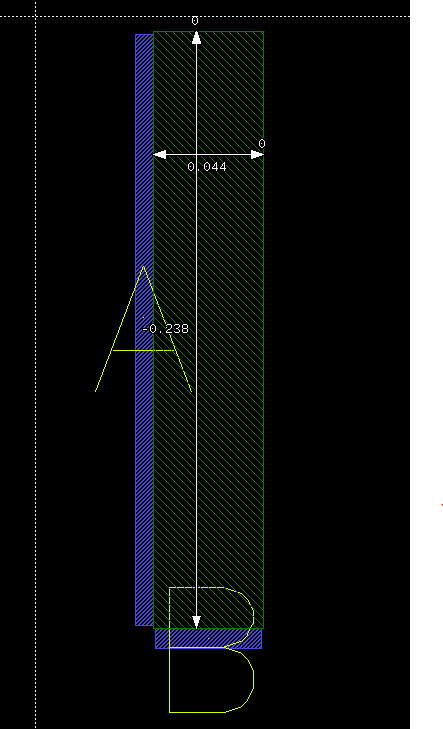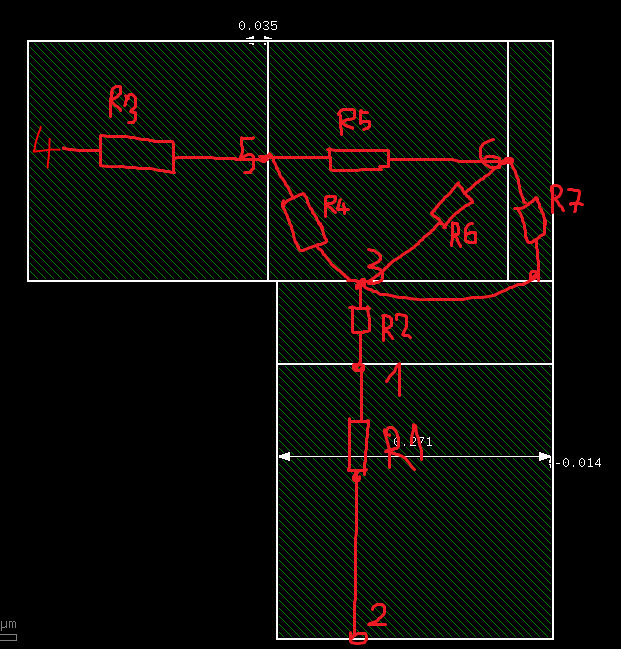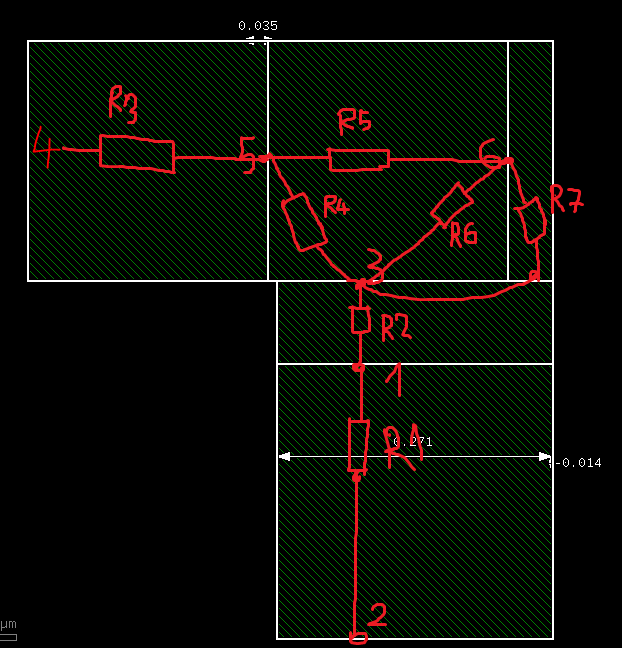# How to specify W,L of resistor

Hello @Matthias ,
I trying to understand How does Klayout specify W, L of resistor in this case, Can you explain?``````r_body = polygons(32, 0)
r_connector = polygons(31,0)
pin = labels(131,0)
connect(r_connector,pin)
extract_devices(resistor("Res",1),{ "R" => r_body,"C" => r_connector })
``````

result:

circuit resistor ();
device Res \$1 (A=B,B=A) (R=1.02877697842,L=0.143,W=0.139,A=0.010472,P=0.564);
end;

Thank you,
dai

• Hello @dai,

So the blue patched are your terminals? That is kind of strange - usually they are connected on opposite sides.

The computation is L and W assumes a rectangular geometry with two ends being entirely assigned to "contact". The computation follows this scheme:

• Take all edges forming the resistor body
• Extract all edges that make the contacts (-> w_edges)
• Subtract w_edges from all edges taking the rest (-> l_edges)

W is then computed as "total_length(w_edges) / 2" and L is computed as "total_length(l_edges) / 2".

In your case this leads to W being the average of the lengths of the blue stripes and L the average of basically nothing and the long path at the top and right side.

Matthias

• Hello @Matthias
Yes, it is terminals.
i trying extract resistor of polygon shape(L,T.. shape), i split polygons to squares and each square is a resistor so it form resistor like that. Here is a example.Thank you so much.
dai

• I see - I guess my approximation is kind of crude for this application, but basically W and L should should give some average path length and width. Approximately.

Matthias

• Hi @Matthias
BTW
Have any function help to calculate resistance from node 5 to node 6 in this picture?. I can not use netlist.simplify Because it combine all resistor to one. I trying to flag where is high resistor compare others.Thank you so much!
dai

• It looks like an over-elaborate version of the old school
"counting squares and corners" approach. For a fully
ortho resistor, seems like you could collect rectangles
(w/ orientation relative to current-flow axis, for squares
calculation apiece) and corners (treated as half-square)
pretty straightforwardly?

Now the contact cartoon makes zero sense, there's a
trivial gap where resistance will be way low irrespective
of bulk sheet resistance and further-out geometry. It
also does not match the latest "squares" figure, in the
contact-location aspect.

• @dick_freebird Looks to me like the approach is to subdivide into pieces with a fixed dimension. So the small portion is a left-over part ...

Matthias

• Thank you, @dick_freebird and @Matthias
I think i have to subdivide net into pieces because my net is complex and layout guys just want to show resistance between two specified points that they can select.

dai

• Design resistors are (almost) always simple rectangles with terminals located on the opposite sides, corner to corner. Then you get a simple resistance formula: R=W/L*rsh.

For complex (non-1D) layouts like this, you need to mesh your area, apply proper boundary conditions, simulate, integrate the current along your ports / boundary, divide voltage by current, to get the resistance.

You can either spend some time doing that, or use a free field solver, or buy a commercial tool.
It's a tradeoff between your urgency, capabilities, money you are willing to spend on this, and guaranteed speed of getting the results and their quality.